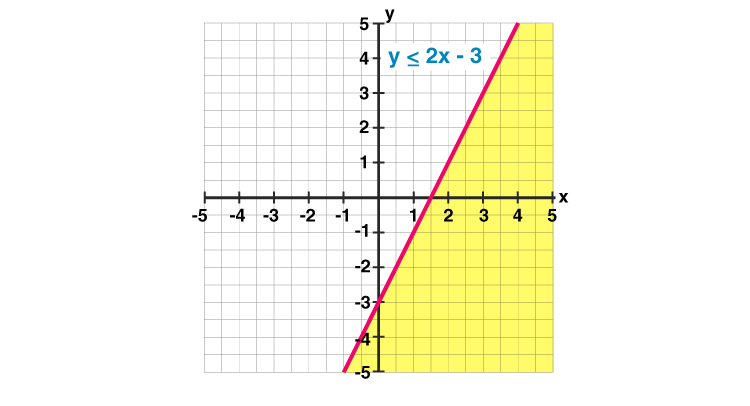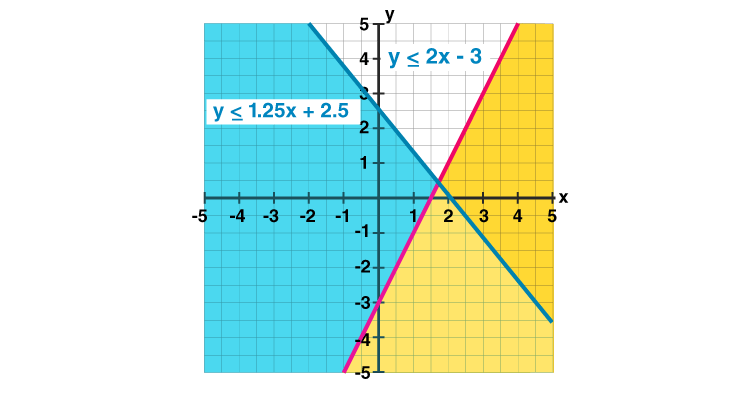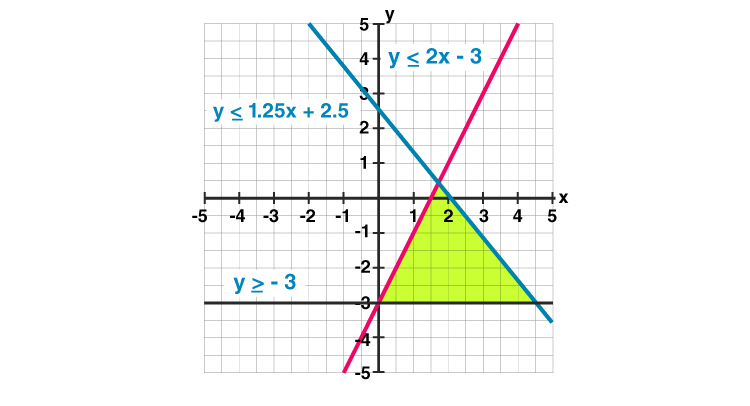# Linear Inequalities

Linear inequalities are the expressions where any two values are compared by the inequality symbols such as, ‘<’, ‘>’, ‘≤’ or ‘≥’. These values could be numerical or algebraic or a combination of both. For example, 10<11, 20>17 are examples of numerical inequalities and x>y, y<19-x, x ≥ z > 11 are the examples of algebraic inequalities (also called literal inequalities). Learn linear inequalities for class 11 here.

The symbols which represent inequalities are:

• < (less than)
• > (greater than)
• ≤ (less than or equal to)
• ≥ (greater than or equal to)
• ≠ (not equal to)

The symbols ‘<‘ and ‘>’ express the strict inequalities and the symbols ‘≤’ and ‘≥’ denote slack inequalities. A linear inequality seems exactly like a linear equation where the inequality sign is replaced by the equality sign.

## Linear Inequalities Graphing

When we plot the graph for inequalities, we can see the graph of an ordinary linear function. But in the case of a linear function, the graph is a line and in case of inequalities, the graph is the area of the coordinate plane that satisfies the inequality.

The linear inequality graph divides the coordinate plane into two parts by a borderline. This line is the line that belongs to the function. One part of the borderline consists of all solutions to the inequality. The borderline is dashed for inequalities ‘>’ and ‘<’ and solid for ‘≥’ and ‘≤’.

To plot an inequality graph, we need to follow three steps:

• Rearrange the given equation such that the variable ‘y’ is on the left-hand side and the rest of the equation on the right-hand side. For example, y>x+2
• Plot the graph for “y=x+2” line by putting the values for x.
• Remember to draw a solid line for y≤ or y≥ and a dashed line for y< or y>.
• Now, shade the line as per inequalities, such as above the line for a “greater than” (y> or y≥) and below the line for a “less than” (y< or y≤).

## System of Linear Inequalities

A system of linear inequalities in two variables includes at least two linear inequalities in the identical variables. When we solve linear inequality then we get an ordered pair. So basically, in a system, the solution to all inequalities and the graph of the linear inequality is the graph displaying all solutions of the system. Let us see an example to understand it.

Example: Graph the system of inequalities

$\left\{\begin{array}{c} y \leq 2 x-3 \\ y \geq-3 \\ y \leq-1.25 x+2.5 \end{array}\right.$

Firstly, we need to plot one line at a time in the same coordinate plane and then shade half of the same coordinate plane that satisfies the inequality condition.The solution area we get by the intersection of the half of planes is presented in a darker shade.Normally, only the solution area is shaded by which we can easily see the solution region.## Linear Inequalities Problems

Question: Solve the inequality 4 ( x + 2 ) − 1 > 5 − 7 ( 4 − x )

Solution: Given,

4 ( x + 2 ) − 1 > 5 − 7 ( 4 − x )

4 x + 8 − 1 > 5 − 28 + 7 x

4 x + 7 > − 23 + 7 x

− 3 x > − 30

x < 10

Hence, x lies between -∞ and less than 10.

Question: Solve the following inequality 8 ≤ 3 − 5 x < 12

Solution: Given,

8 ≤ 3 − 5 x < 12

5 ≤ − 5 x < 9

− 1 ≥ x > − 9/5

Hence, x lies between the interval (-9/5,-1).